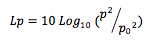# What Is a Decibel?

The sound pressure level (Lp) in decibels (dB) is given by:Where:

p = (rms) pressure of sound level being measured

p0 = (rms) reference pressure of 20μ Pa (approximate threshold of hearing)

However, decibels are also used to measure sound intensity and sound power, because they behave in the same way as the sound pressure level (these are all different aspects of the same phenomenon). In these cases the ratios are of Watts per square metre (Wm-2) and Watts respectively.

Unfortunately, the fact that dB can refer to these different parameters can cause considerable confusion and has led to significant errors on occasion.